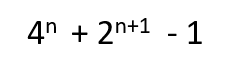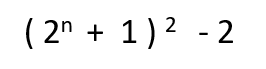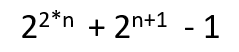# Program to find the nth Kynea number

Given a positive integer n, the task is to find nth Kynea number.

Kynea number: In mathematics, a Kynea number is a positive integer of the form:where n is a positive integer.

The equivalent formula for nth Kynea number is:The first few Kynea number are:

7, 23, 79, 287, 1087, 4223, 16639, 66047, 263167, 1050623, 4198399, …..

Examples:

```Input: 2
Output: 23
Putting n = 2 in formula,
= 42 + 2 2+1 - 1
= 16 + 8 -1
= 23
```

Method 1: A Simple Solution is to find out nth number by putting the value of n in the formulaBelow is the implementation of above approach:

## C++

 `// CPP code to find nth Kynea number ` ` `  `#include ` `using` `namespace` `std; ` ` `  `// Function to calculate nth kynea number ` `long` `nthKyneaNumber(``int` `n) ` `{ ` ` `  `    ``// Calculate nth kynea number ` `    ``// using formula ((2^n + 1)^2 ) -2 ` ` `  `    ``// Firstly calculate 2^n + 1 ` `    ``n = (1 << n) + 1; ` ` `  `    ``// Now calculate (2^n + 1)^2 ` `    ``n = n * n; ` ` `  `    ``// Now calculate ((2^n + 1)^2 ) - 2 ` `    ``n = n - 2; ` ` `  `    ``// return nth Kynea number ` `    ``return` `n; ` `} ` ` `  `// Driver Program ` `int` `main() ` `{ ` `    ``int` `n = 8; ` ` `  `    ``// print nth kynea number ` `    ``cout << nthKyneaNumber(n); ` ` `  `    ``return` `0; ` `} `

## Java

 `// JAVA code to find nth Kynea number ` ` `  `class` `GFG { ` ` `  `    ``// Function to calculate nth kynea number ` `    ``static` `long` `nthKyneaNumber(``int` `n) ` `    ``{ ` ` `  `        ``// Calculate nth kynea number ` `        ``// using formula ((2^n + 1)^2 ) -2 ` ` `  `        ``// Firstly calculate 2^n + 1 ` `        ``n = (``1` `<< n) + ``1``; ` ` `  `        ``// Now calculate (2^n + 1)^2 ` `        ``n = n * n; ` ` `  `        ``// Now calculate ((2^n + 1)^2 ) - 2 ` `        ``n = n - ``2``; ` ` `  `        ``// return nth Kynea number ` `        ``return` `n; ` `    ``} ` ` `  `    ``// Driver Program ` `    ``public` `static` `void` `main(String[] args) ` `    ``{ ` `        ``int` `n = ``2``; ` ` `  `        ``// print nth kynea number ` `        ``System.out.println(nthKyneaNumber(n)); ` `    ``} ` `} `

## Python

 `# Python code to find nth Kynea number ` ` `  `# Function to calculate nth kynea number  ` `def` `nthKyneaNumber( n):  ` `     `  `    ``# Calculate nth kynea number ` `    ``# using formula ((2 ^ n + 1)^2 ) -2 ` `     `  `    ``# Firstly calculate 2 ^ n + 1 ` `    ``n ``=` `( ``1` `<< n) ``+` `1` `     `  `    ``# Now calculate (2 ^ n + 1)^2  ` `    ``n ``=` `n ``*` `n ` `     `  `    ``# Now calculate ((2 ^ n + 1)^2 ) - 2 ` `    ``n ``=` `n``-``2` `     `  `     `  `    ``# return nth Kynea number ` `    ``return` `n ` `     `  ` `  ` `  `# Driver Code  ` `n ``=` `2`  ` `  `# print nth kynea number ` `print``(nthKyneaNumber(n)) `

## C#

 `// C# code to find nth Kynea number ` ` `  `using` `System; ` `class` `GFG { ` ` `  `    ``// Function to calculate nth kynea number ` `    ``static` `long` `nthKyneaNumber(``int` `n) ` `    ``{ ` ` `  `        ``// Calculate nth kynea number ` `        ``// using formula ((2^n + 1)^2 ) -2 ` ` `  `        ``// Firstly calculate 2^n + 1 ` `        ``n = (1 << n) + 1; ` ` `  `        ``// Now calculate (2^n + 1)^2 ` `        ``n = n * n; ` ` `  `        ``// Now calculate ((2^n + 1)^2 ) - 2 ` `        ``n = n - 2; ` ` `  `        ``// return nth Kynea number ` `        ``return` `n; ` `    ``} ` ` `  `    ``// Driver Program ` `    ``public` `static` `void` `Main() ` `    ``{ ` `        ``int` `n = 2; ` ` `  `        ``// print nth kynea number ` `        ``Console.WriteLine(nthKyneaNumber(n)); ` `    ``} ` `} `

## PHP

 `

Output:

```66047
```

Method 2: This solution is based on the fact that every Kynea number follows a specific pattern in their binary representation. nth Kynea number can be represented in binary as a single leading one followed by exactly n-1 consecutive 0’s, followed by n+1 consecutive 1’s.

Example:

```23 is 2nd kynea number
It can be represented in binary as 10111
(Single leading one, followed by n - 1 ( i.e 2-1=1 ) consecutive 0's,
followed by n + 1 ( i.e 2 + 1 = 3 ) consecutive 1's.)
```
n nth Kynea Number Binary Representation
1 7 111
2 23 10111
3 79 1001111
4 287 100011111
5 1087 10000111111
6 4223 1000001111111

Observing the binary pattern of Kynea number in above table, the nth Kynea number can be easily calculated using the formula:Example:

```Input: n = 3
Output: 79
Using formula,
= 26 + 24 -1
= 64 + 15
= 79
```

Below is the implementation of above approach

## C++

 `// CPP code to find nth Kynea number ` ` `  `#include ` `using` `namespace` `std; ` ` `  `// Function to calculate nth kynea number ` `long` `nthKyneaNumber(``int` `n) ` `{ ` ` `  `    ``// Calculate nth kynea number ` `    ``return` `((1 << (2 * n)) + (1 << (n + 1)) - 1); ` `} ` ` `  `// Driver Program ` `int` `main() ` `{ ` `    ``int` `n = 2; ` ` `  `    ``// print nth kynea number ` `    ``cout << nthKyneaNumber(n); ` ` `  `    ``return` `0; ` `} `

## Java

 `// JAVA code to find nth Kynea number ` ` `  `class` `GFG { ` ` `  `    ``// Function to calculate nth kynea number ` `    ``static` `long` `nthKyneaNumber(``int` `n) ` `    ``{ ` ` `  `        ``// Calculate nth kynea number ` `        ``return` `((``1` `<< (``2` `* n)) + (``1` `<< (n + ``1``)) - ``1``); ` `    ``} ` ` `  `    ``// Driver Program ` `    ``public` `static` `void` `main(String[] args) ` `    ``{ ` `        ``int` `n = ``2``; ` ` `  `        ``// print nth kynea number ` `        ``System.out.println(nthKyneaNumber(n)); ` `    ``} ` `} `

## Python

 `# Python code to find nth Kynea number ` ` `  `# Function to calculate nth kynea number  ` `def` `nthKyneaNumber( n):  ` ` `  `    ``# Calculate nth kynea number     ` `    ``return` `(( ``1` `<< (``2` `*` `n)) ``+` `( ``1` `<< (n ``+` `1``)) ``-``1` `)  ` `     `  ` `  `# Driver Code  ` `n ``=` `2`  ` `  `# print nth kynea number ` `print``(nthKyneaNumber(n)) `

## C#

 `// C# code to find nth Kynea number ` ` `  `using` `System; ` `class` `GFG { ` ` `  `    ``// Function to calculate nth kynea number ` `    ``static` `long` `nthKyneaNumber(``int` `n) ` `    ``{ ` ` `  `        ``// Calculate nth kynea number ` `        ``return` `((1 << (2 * n)) + (1 << (n + 1)) - 1); ` `    ``} ` ` `  `    ``// Driver Program ` `    ``public` `static` `void` `Main() ` `    ``{ ` `        ``int` `n = 2; ` ` `  `        ``// print nth kynea number ` `        ``Console.WriteLine(nthKyneaNumber(n)); ` `    ``} ` `} `

## PHP

 ` `

Output:

```23
```

Attention reader! Don’t stop learning now. Get hold of all the important DSA concepts with the DSA Self Paced Course at a student-friendly price and become industry ready.

My Personal Notes arrow_drop_upCheck out this Author's contributed articles.

If you like GeeksforGeeks and would like to contribute, you can also write an article using contribute.geeksforgeeks.org or mail your article to contribute@geeksforgeeks.org. See your article appearing on the GeeksforGeeks main page and help other Geeks.

Please Improve this article if you find anything incorrect by clicking on the "Improve Article" button below.

Improved By : vt_m, Akanksha_Rai, nidhi_biet